Next: Tidal elongation Up: Rotating reference frames Previous: Coriolis force

# Rotational flattening

Consider the equilibrium configuration of a self-gravitating celestial body, composed of incompressible fluid, that is rotating steadily and uniformly about some fixed axis passing through its center of mass. Let us assume that the outer boundary of the body is spheroidal. (See Section 3.6.) Let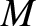be the body's total mass,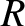its mean radius,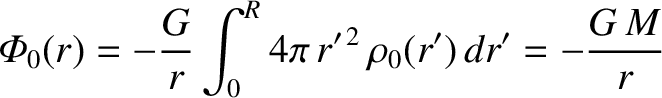its ellipticity, and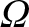its angular rotation velocity. Suppose, finally, that the body's axis of rotation coincides with its axis of symmetry, which is assumed to run along the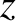-axis.

Let us transform to a non-inertial frame of reference that co-rotates with the body about the-axis, and in which the body consequently appears to be stationary. From Section 6.3, the problem is now analogous to that of a non-rotating body, except that the acceleration is written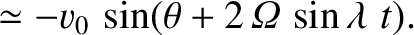, where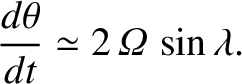is the gravitational acceleration,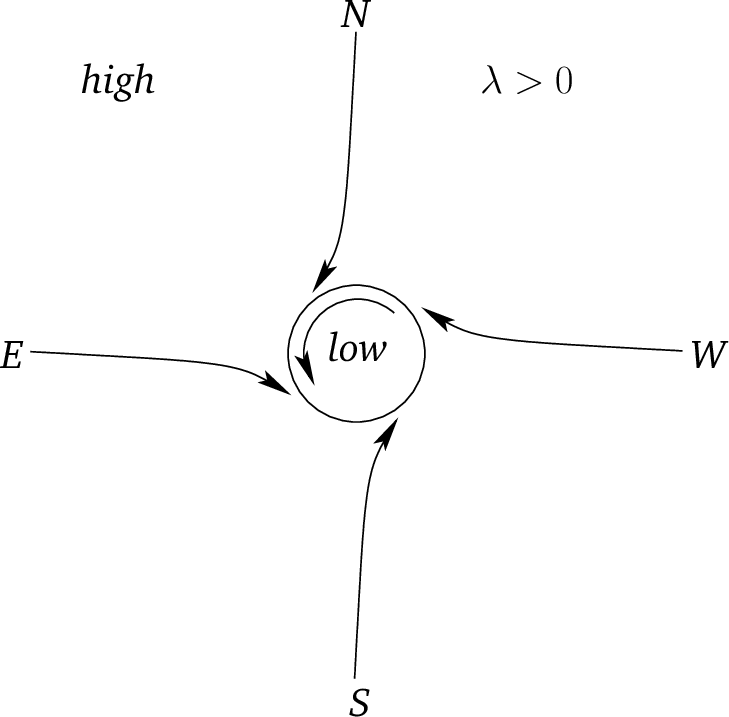the centrifugal acceleration, and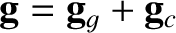the gravitational potential. The latter acceleration is of magnitude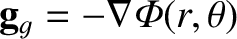and is everywhere directed away from the axis of rotation. (See Section 6.3.) Here,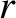and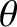are spherical coordinates whose origin is the body's geometric center and whose symmetry axis coincides with the axis of rotation. The centrifugal acceleration is thus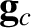(6.33)

It follows that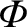, where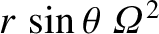(6.34)

can be thought of as a sort of centrifugal potential. Thus, the total acceleration is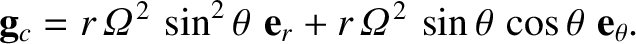(6.35)

It is convenient to write the centrifugal potential in the form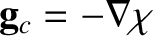(6.36)

where the dimensionless parameter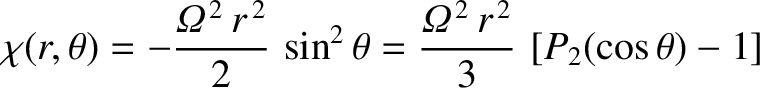(6.37)

is the typical ratio of the centrifugal acceleration to the gravitational acceleration at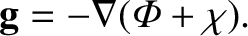. Let us assume that this ratio is small; that is,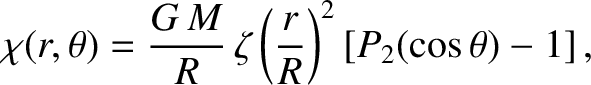.

As before (see Section 3.6), the criterion for an equilibrium state is that the total potential be uniform over the body's surface, so as to eliminate any tangential forces that cannot be balanced by internal pressure. Let us assume that the surface satisfies [see Equation (3.56)]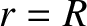(6.38)

where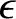(6.39)

Here,is the body's mean radius,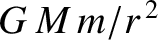the radius at the poles (i.e., along the axis of rotation), and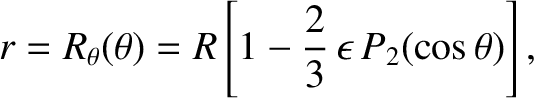the radius at the equator (i.e., perpendicular to the axis of rotation). (See Figure 6.4.) It is assumed that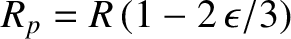, so that the body is almost spherical. The external (to the body) gravitational potential can be written [see Equation (3.66)]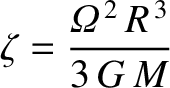(6.40)

where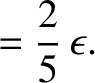. The equilibrium configuration is specified by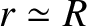(6.41)

where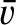is a constant. It follows from Equations (6.36), (6.38), and (6.40) that, to first order inand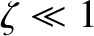,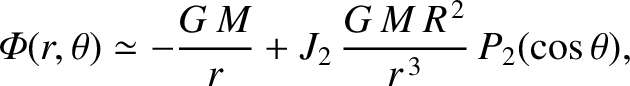(6.42)

which yields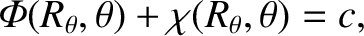(6.43)

For the special case of a uniform-density body, we have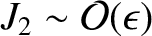. [See Equation (3.64).] Hence, the previous equation simplifies to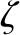(6.44)

or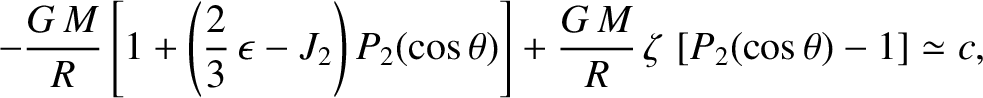(6.45)

We conclude, from the preceding expression, that the equilibrium configuration of a (relatively slowly) rotating self-gravitating fluid mass is an oblate spheroid--a sphere that is slightly flattened along its axis of rotation. The degree of flattening is proportional to the square of the rotation rate.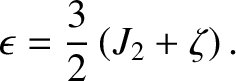The result of Equation (6.44) was derived on the assumption that there is zero shear stress at the surface of a uniform-density, rotating, self-gravitating, celestial body. This is certainly true for a fluid body, because fluids (by definition) are unable to withstand shear stresses. Solids, on the other hand, can withstand such stresses to a limited extent. Hence, it is not necessarily true that there is zero shear stress at the surface of a solid rotating body, such as the Earth. Let us investigate whether expression (6.44) needs to be modified for such a body.

In the presence of the centrifugal potential specified in Equation (6.36), the normal elastic (i.e., non-hydrostatic) stress acting (outward) across the surface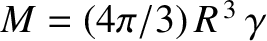, for a body of mean radius, ellipticity, and uniform density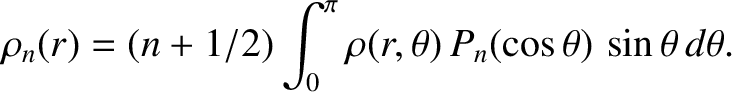, can be written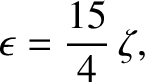, where [see Equation (C.56)]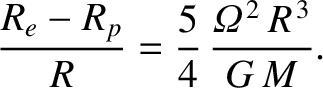(6.46)

and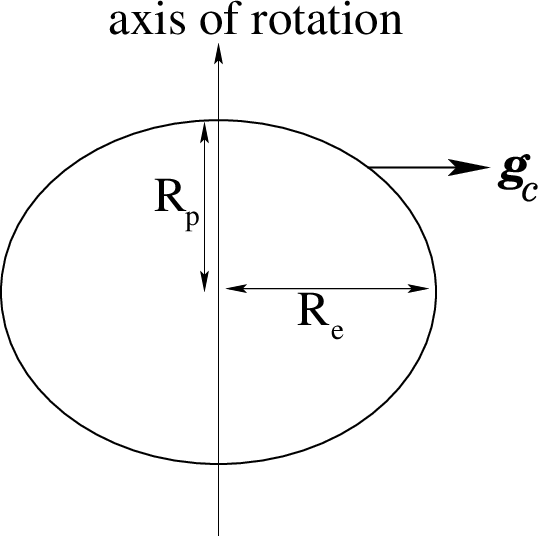is the yield stress of the material from which the body is composed (i.e., the critical shear stress above which the material flows like a liquid) (Love 2011). The shear stress is proportional to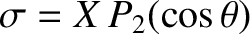. Furthermore,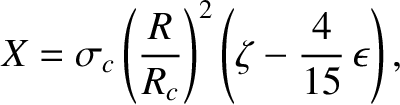(6.47)

For the rock that makes up the Earth's mantle,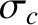and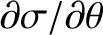, giving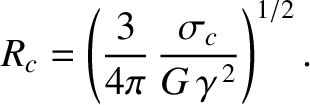(de Pater and Lissauer 2010.) Let us assume that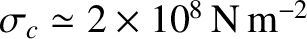, which implies that, in the absence of the centrifugal potential, the self-gravity of the body in question is sufficiently strong to force it to adopt a spherical shape. (See Section 3.6.) If the surface shear stress is less than the yield stress (i.e., if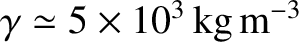) then the body responds elastically to the stress in such a manner that [see Equation (C.57)]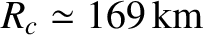(6.48)

where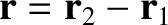is the shear modulus, or rigidity, of the body's constituent material (Love 2011). For the rock that makes up the Earth's mantle,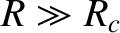(de Pater and Lissauer 2010). It follows that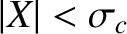(6.49)

where [see Equation (C.58)]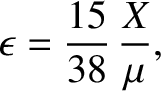(6.50)

is a dimensionless quantity that is termed the body's effective rigidity. On the other hand, if the surface shear stress is greater than the yield stress then the body flows like a liquid until the stress becomes zero. So, it follows from Equation (6.46) that(6.51)

which is identical to Equation (6.44). Hence, we deduce that the rotational flattening of a solid, uniform-density, celestial body is governed by Equation (6.49) if the surface shear stress does not exceed the yield stress, and by Equation (6.44) otherwise. In the former case, the conditionis equivalent to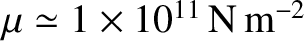, where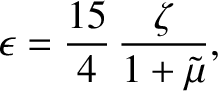(6.52)

For the rock that makes up the Earth's mantle [for which,,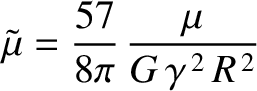, and(de Pater and Lissauer 2010)], we find that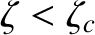(6.53)

Thus, ifthen the rotational flattening of a uniform body made up of such rock is governed by Equation (6.49), but if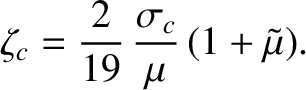then the flattening is governed by Equation (6.51).

Now, for the case of the Earth itself,,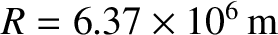, and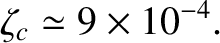(Yoder 1995). It follows that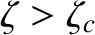(6.54)

Because, we deduce that the Earth's centrifugal potential is sufficiently strong to force its constituent rock to flow like a liquid. Hence, the rotation flattening is governed by Equation (6.51), which implies that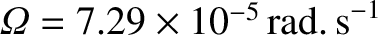(6.55)

This corresponds to a difference between the Earth's equatorial and polar radii of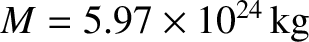(6.56)

In fact, the observed degree of rotational flattening of the Earth is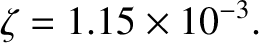(Yoder 1995), corresponding to a difference between equatorial and polar radii of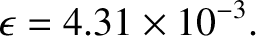. Our analysis has overestimated the Earth's rotational flattening because, for the sake of simplicity, we assumed that the terrestrial interior is of uniform density. In reality, the Earth's core is significantly denser than its crust. (See Section D.6.) Incidentally, the observed value of the parameter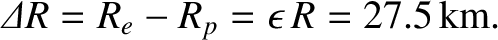, which measures the strength of the Earth's quadrupole gravitational field, is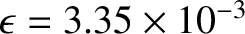(Yoder 1995). Hence,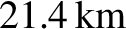. In other words, the Earth's rotational flattening satisfies Equation (6.43) extremely accurately. This confirms that although the Earth is not a uniform-density body its response to the centrifugal potential is indeed fluid-like [because Equation (6.43) was derived on the assumption that the surface of the rotating body in question is in hydrostatic equilibrium in the co-rotating frame].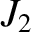For the planet Jupiter,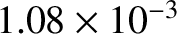,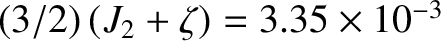, and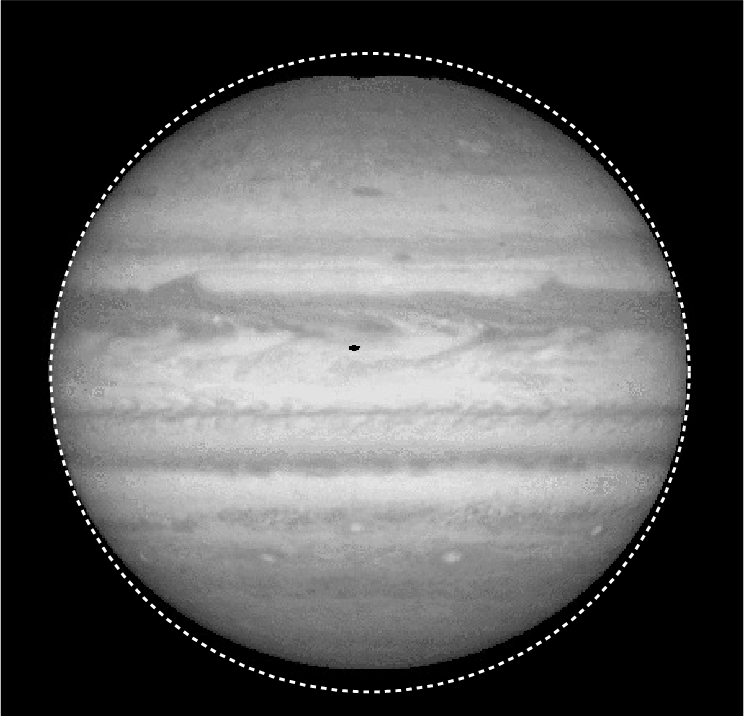(Yoder 1995; Seidelmann et al. 2007). Hence,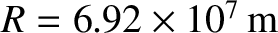(6.57)

Because Jupiter is largely composed of liquid, its rotation flattening is governed by Equation (6.51), which yields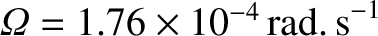(6.58)

This degree of flattening is much larger than that of the Earth, owing to Jupiter's relatively large radius (about ten times that of Earth), combined with its relatively short rotation period (about 0.4 days). In fact, the rotational flattening of Jupiter is clearly apparent from images of this planet. (See Figure 6.5.) The observed degree of rotational flattening of Jupiter is actually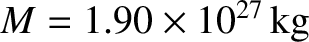(Yoder 1995). Our estimate foris slightly too large because Jupiter has a mass distribution that is strongly concentrated at its core. (See Section D.6.) Incidentally, the measured value offor Jupiter is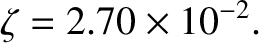(Yoder 1995). Hence,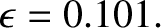. Thus, Jupiter's rotational flattening also satisfies Equation (6.43) fairly accurately, confirming that its response to the centrifugal potential is fluid-like.Next: Tidal elongation Up: Rotating reference frames Previous: Coriolis force
Richard Fitzpatrick 2016-03-31